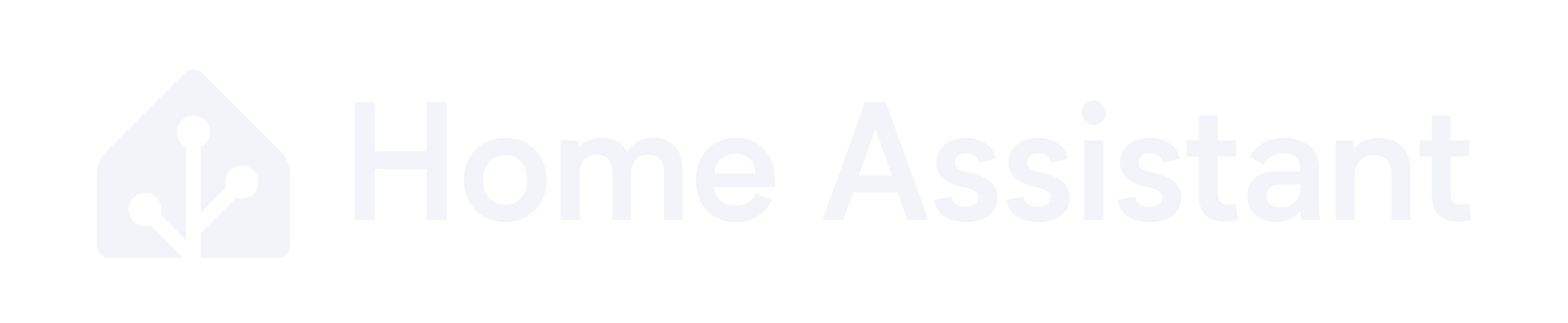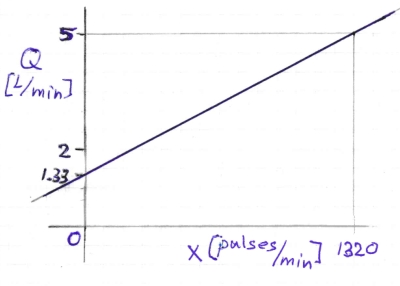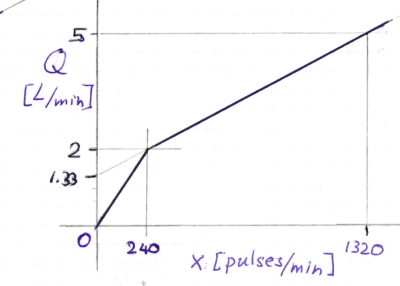# Calibration settings for YF-B10 pulse counter?

Hi All
Has somebody ever managed to calibrate this sensor the right way ?

With the following recomended function on another thread, I get crazy values (500 L when the tap is opened for 2 seconds).

``````  - lambda: return x * (6 * x - 8);
``````

I found another function based on the manufacturer characteristics ( F = (6 x Q - 8)) but same here, the reading is not accurate.

I can fill 1L in about 15 to 20 seconds with 373 pulses.

Can someone help ?

Thanks !

I don’t have such a flow meter myself, so cannot test this, but as I understand it, in this formula F stands for Frequency in [pulses/second] and Q stands for Flow in [liters/minute].
As you want to determine the flow, with the pulse count as input, you have to change the formula like this:
Q = (F + 8) / 6 with F in [pulses/sec] and Q in [L/min]
However, the ESPHome Pulse Counter Sensor defaults to [pulses/minute], so you need to modify the formula like this (converting for [pulses/sec]):
Q = ((F / 60) + 8) / 6 with F in [pulses/min] and Q in [L/min]
So the pulse_counter filter then should be:

``````filters:
- lambda: return (((x / 60) + 8) / 6);
``````

Does that make sense?
If the result is still inaccurate this Github post might be of interest: FY-B10 or YF-B10 flow sensor ESP32 example

1 Like

Hi - I just gave a look and the result seems to be better, closer to the reality.
Will dig into it this evening. Thanks !

So the pulse_counter filter then should be:

``````filters:
- lambda: return (((x / 60) + 8) / 6);
``````

Does that make sense?

Ok, so far with a flow meter test with 3L of water, the sensor seems to provide accurate results, so many thanks ! What I don’t know now is how to change the default value (when the tap is closed and X = 0). It returns 1.33 L / min.

Any idea ?

Ah! Of course, I didn’t think of that.
When x = 0 then Q = (((0 / 60) + 8) / 6 = 8 / 6 = 1.333 and that should of course be 0 as well.
It now is in fact a linear function like this, with Q not reaching 0 when x is 0:This type of sensor has a minimum useable flow rate.
One option to solve this issue is to add a step in the function that assures the flow rate to go to zero when the rate is below the minimum useable flow rate. Something like this:While searching the Internet I find several different versions of this YF-B10 sensor with a flow rate range of 2 to 50 L/minute.
So when we assume the minimum useable flow rate to be Q = 2 L/min then the minimum useable pulse rate will be x = 240 pulses/min
So the pulse counter filter can be modified like this to assure the flow rate will go to zero when the pulse count is below 240 pulses/min:

``````filters:
- lambda: !lambda |-
if (x < 240) return (x / 120);
return (((x / 60) + 8) / 6);
``````
2 Likes

Awesome and brilliant, thanks !!